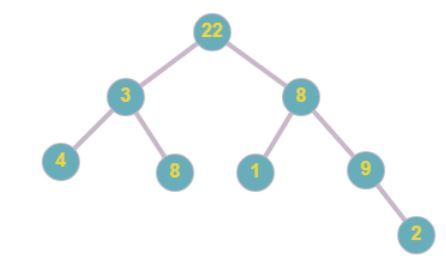New update is available. Click here to update.

# NINJA GRANDPARENTS

Last Updated: 27 Mar, 2021
Difficulty: Easy

## PROBLEM STATEMENT

#### Example :

``````Consider the following binary tree :
````````````So the sum of child nodes having even valued grandparents is ‘4 + 8 + 1 + 9 + 2 = 24’
``````

#### Input Format :

``````The first line contains an integer ‘T' which denotes the number of test cases to be run. Then the test cases follow.
The first line of each test case contains the tree elements in the level order form separated by a single space.
If any node does not have a left or right child, take -1 in its place. Refer to the example below.

Example :

Elements are in the level order form. The input consists of values of nodes separated by a single space in a single line. In case a node is null, we take -1 in its place.

For example, the input for the tree depicted in the below image would be :
````````````1
2 3
4 -1 5 6
-1 7 -1 -1 -1 -1
-1 -1
``````

#### Explanation :

``````Level 1 :
The root node of the tree is 1

Level 2 :
Left child of 1 = 2
Right child of 1 = 3

Level 3 :
Left child of 2 = 4
Right child of 2 = null (-1)
Left child of 3 = 5
Right child of 3 = 6

Level 4 :
Left child of 4 = null (-1)
Right child of 4 = 7
Left child of 5 = null (-1)
Right child of 5 = null (-1)
Left child of 6 = null (-1)
Right child of 6 = null (-1)

Level 5 :
Left child of 7 = null (-1)
Right child of 7 = null (-1)

The first not-null node (of the previous level) is treated as the parent of the first two nodes of the current level. The second not-null node (of the previous level) is treated as the parent node for the next two nodes of the current level and so on.

The input ends when all nodes at the last level are null (-1).
``````
##### Note :
``````The above format was just to provide clarity on how the input is formed for a given tree.

The sequence will be put together in a single line separated by a single space. Hence, for the above-depicted tree, the input will be given as:

1 2 3 4 -1 5 6 -1 7 -1 -1 -1 -1 -1 -1
``````

#### Output Format :

``````For each test case, print the sum of all child nodes having even valued grandparent nodes.

Print output of each test case in a separate line.
``````

#### Note :

``````You are not required to print anything. It has already been taken care of. Just implement the function.
``````

#### Constraints :

``````1 <= T <= 100
1 <= N <= 3000
0 <= data <= 10^9

Where ‘T’ is the total number of test cases, ‘N’ is the number of nodes of the tree, and ‘data’ is the integer value mentioned on the node.

Time Limit : 1 sec
``````## Approach 1

So the idea here is to traverse the tree and for each node, we check if there is a grandparent that exists for that particular node or not. If it exists we check if it is even or not.

## Algorithm:

• So we use the recursive method for calculating the sum. For this, we declare three variables previous which store the previous node, grandparent which stores information about the grandparent node.
• Now we start traversing our tree with the help of recursion first we call our recursion for the left subtree then for the right subtree.
• And we update or current with node left or right depends on in which direction we are going and we update our previous with current and grandparent with previous.
• Now we check if grandparent exists we further check if it is even we stored the sum of the current node else we recur further.
• In this way, we arrive at our final sum when the recursive call ends or we can say our current becomes NULL.